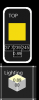# GMLGetting Weird Behavior in a Widget

E

#### Ephemeral

##### Guest
I've been staring at this code for an hour and I need a fresh set of eyes.

I made a color-picker widget for a thing. It looks like this, and it works by clicking on the number you want to change and dragging either left or right. It all works too, except for this one weird bug I can't figure out. For some reason, clicking on either the saturation box or the value box and changing the value causes the values to the left (IE earlier in the for loop) to also drift by some much smaller amount, and I can't figure out why.(Ignore the borked niceslice...)

As you can see, the Hue box is not even showing an integer, which should be impossible since the only place I'm setting any of those three numbers it is through a round() function.
Code:
``````// Get Color Components from Light Color
cpp_color_component = color_get_hue(light_color[active_face]);
cpp_color_component = color_get_saturation(light_color[active_face]);
cpp_color_component = color_get_value(light_color[active_face]);

// Adjust the Value of One Input Field
if (mouse_check_button_pressed(mb_left))
{
// The HSVs
var ll, tt, rr, bb;
var found_input = false;
for (var box = 0; box < 3; box += 1)
{
ll = cpp_input_field_x + (cpp_input_field_width * box);
tt = cpp_input_field_y;
rr = ll + cpp_input_field_width;
bb = cpp_input_field_y + cpp_input_field_height;
if (mouse_x > ll) and (mouse_y > tt) and (mouse_x < rr) and (mouse_y < bb)
{
cpp_active_box = box;
cpp_mouse_start = mouse_x;
found_input = true;
cpp_value_to_modify = cpp_color_component[box];
}
}
// The Brightness
if not (found_input)
{
ll = cpp_center - (cpp_input_field_width / 2);
tt = cpp_input_field_y + cpp_input_field_height;
rr = ll + cpp_input_field_width;
bb = tt + cpp_input_field_height;
if (mouse_x > ll) and (mouse_y > tt) and (mouse_x < rr) and (mouse_y < bb)
{
cpp_active_box = 3;
cpp_mouse_start = mouse_x;
found_input = true;
cpp_value_to_modify = brightness[active_face];
}
}
}
if not (cpp_active_box == noone) // If a Box is Active
{
if (mouse_check_button(mb_left))
{
var difference = mouse_x - cpp_mouse_start;
if (cpp_active_box == 3)
{
brightness[active_face] = clamp(cpp_value_to_modify + (difference / 255), 0, 1);
}
else
{
cpp_color_component[cpp_active_box] = round(clamp(cpp_value_to_modify + difference, 0, 255));
light_color[active_face] = make_color_hsv(cpp_color_component, cpp_color_component, cpp_color_component);
}
}
else // Quit This Input Box and Reset
{
cpp_active_box = noone;
cpp_value_to_modify = 0;
}
}``````
Help?